# Shift Micro-Operations in Computer Architecture

Shift micro-operations are those micro-operations that are used for the serial transfer of information. These are also used in conjunction with arithmetic micro-operation, logic micro-operation, and other data-processing operations. There are three types of shift micro-operations: 1.

## 1. Logical Shift:

It transfers the 0 zero through the serial input. We use the symbols ‘<<‘ for the logical left shift and  ‘>>‘ for the logical right shift.

### Logical Left Shift:

In this shift, one position moves each bit to the left one by one. The Empty least significant bit (LSB) is filled with zero (i.e, the serial input), and the most significant bit (MSB) is rejected.

The left shift operator is denoted by the double left arrow key (<<). The general syntax for the left shift is shift-expression << k.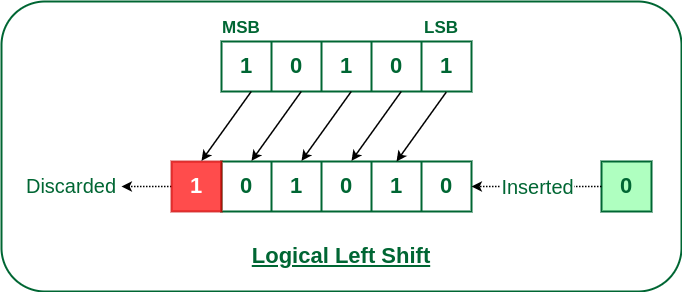Logical Left Shift

Note: Every time we shift a number towards the left by 1 bit it multiplies that number by 2.

### Logical Right Shift

In this shift, each bit moves to the right one by one and the least significant bit(LSB) is rejected and the empty MSB is filled with zero.

The right shift operator is denoted by the double right arrow key (>>). The general syntax for the right shift is “shift-expression >> k”.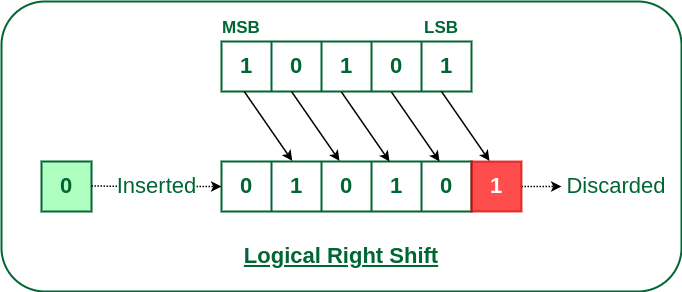Logical Right Shift

Note: Every time we shift a number towards the right by 1 bit it divides that number by 2.

## 2. Arithmetic Shift:

The arithmetic shift micro-operation moves the signed binary number either to the left or to the right position.
Following are the two ways to perform the arithmetic shift.

1. Arithmetic Left Shift
2. Arithmetic Right Shift

### Arithmetic Left Shift:

In this shift, each bit is moved to the left one by one. The empty least significant bit (LSB) is filled with zero and the most significant bit (MSB) is rejected. Same as the Left Logical Shift.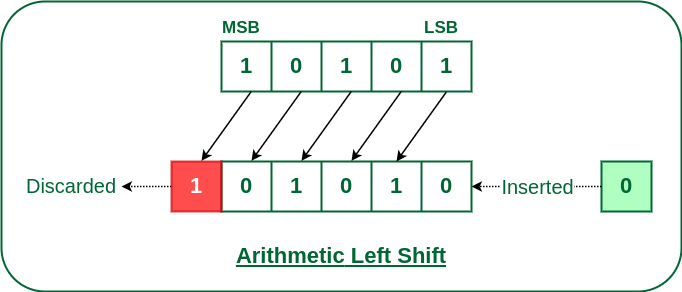Arithmetic Left Shift

### Arithmetic Right Shift:

In this shift, each bit is moved to the right one by one and the least significant(LSB) bit is rejected and the empty most significant bit(MSB) is filled with the value of the previous MSB.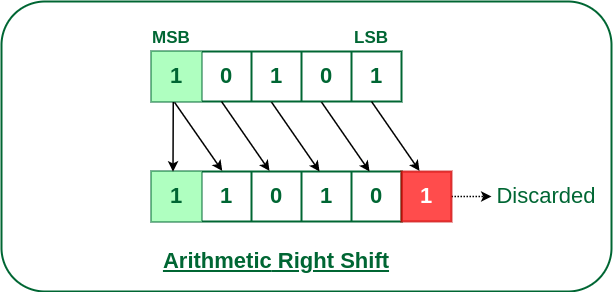Arithmetic Right Shift

## 3. Circular Shift:

The circular shift circulates the bits in the sequence of the register around both ends without any loss of information.
Following are the two ways to perform the circular shift.

1. Circular Shift Left
2. Circular Shift Right

### Circular Left Shift:

In this micro shift operation each bit in the register is shifted to the left one by one. After shifting, the LSB becomes empty, so the value of the MSB is filled in there.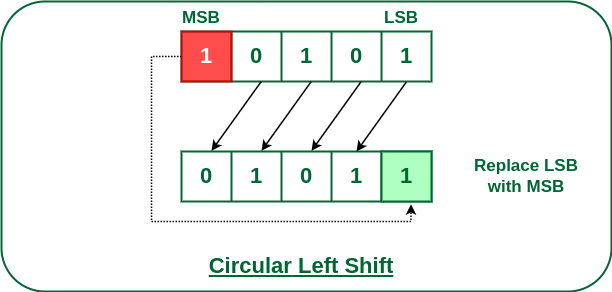Circular Left Shift

### Circular Right Shift:

In this micro shift operation each bit in the register is shifted to the right one by one. After shifting, the MSB becomes empty, so the value of the LSB is filled in there.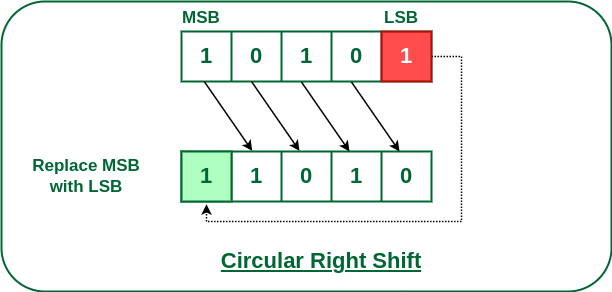Circular Right Shift